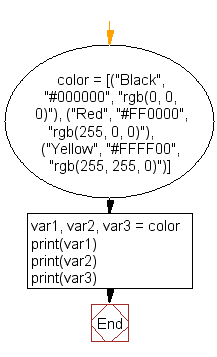﻿ Python: Split a list into different variables - w3resource# Python: Split a list into different variables

## Python List: Exercise - 43 with Solution

Write a Python program to split a list into different variables.

Sample Solution:-

Python Code:

``````color = [("Black", "#000000", "rgb(0, 0, 0)"), ("Red", "#FF0000", "rgb(255, 0, 0)"),
("Yellow", "#FFFF00", "rgb(255, 255, 0)")]
var1, var2, var3 = color
print(var1)
print(var2)
print(var3)
```
```

Sample Output:

```('Black', '#000000', 'rgb(0, 0, 0)')
('Red', '#FF0000', 'rgb(255, 0, 0)')
('Yellow', '#FFFF00', 'rgb(255, 255, 0)')
```

Pictorial Presentation:Flowchart:## Visualize Python code execution:

The following tool visualize what the computer is doing step-by-step as it executes the said program:

Python Code Editor:

Have another way to solve this solution? Contribute your code (and comments) through Disqus.

What is the difficulty level of this exercise?

Test your Python skills with w3resource's quiz

﻿

## Python: Tips of the Day

Floor Division:

When we speak of division we normally mean (/) float division operator, this will give a precise result in float format with decimals.

For a rounded integer result there is (//) floor division operator in Python. Floor division will only give integer results that are round numbers.

```print(1000 // 300)
print(1000 / 300)```

Output:

```3
3.3333333333333335```